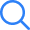# Fluid Mechanics...

TIME LEFT -
• ###### Question 1
2 / -0.33

When a dolphin glides through air, it experiences an external pressure of 0.75 m of mercury. The absolute pressure on dolphin when it is 5 m below the free surface of the water is

• ###### Question 2
2 / -0.33

Calculate the capillary rise or fall in a glass tube of 2.5 mm diameter when immersed vertically in mercury. Take surface tension σ = 0.52 N/m for mercury in constant with glass and angle of contact = 130°

• ###### Question 3
2 / -0.33

Velocity profile over a curved surface is given as : $$\frac{u}{U} = 2{\left( {\frac{y}{\delta }} \right)^2} - {\left( {\frac{y}{\delta }} \right)^3}$$

The flow ______

• ###### Question 4
2 / -0.33

The value of friction factor is misjudged by 25% in using Darcy-Weisbach equation. The resulting error in the discharge will be?

• ###### Question 5
2 / -0.33

A centrifugal pump lifts 0.0125 m3 / s of water from a well with a static lift of 30 m. If the brake power of the driving electric motor is 5 kW, what is the overall efficiency of the pump-set?

• ###### Question 6
2 / -0.33

Which of the following conditions is true regarding the validity of Pascal’s Law?

I- It is valid for ideal fluid in motion.

II- It is valid when real fluids with rigid body motion.

• ###### Question 7
2 / -0.33

A head loss in 100 m length of a 0.1 m diameter pipe (f=0.02) carrying water is 10 m. The boundary shear stress in Pa is ____

• ###### Question 8
2 / -0.33

Which of the following represents generalised continuity equation?

• ###### Question 9
2 / -0.33

In an orifice meter, coefficient of discharge is 0.58 and coefficient of vena contracta is 0.61, what is coefficient of velocity?

• ###### Question 10
2 / -0.33

In a flow over a flat plate, laminar boundary layer exists where velocity distribution is

$$\frac{u}{U} = 0.5\frac{y}{\delta }$$

Ratio of momentum thickness to boundary layer thickness is____

• ###### Question 11
2 / -0.33

Match the following:

 P Reynold’s model i. Cavitation Q Froude model ii. Capillary rise R Euler model iii. Pipe flow S Weber model iv. Flow over spillways

• ###### Question 12
2 / -0.33

A 1 : 6 scale model of a passenger car is tested in a wind tunnel. The prototype velocity is 60 km/hr. The model drag is 250 N. The air in the model and prototype can be assumed to have the same properties. Which of the following statements are true?

• ###### Question 13
2 / -0.33

A smooth flat plate of total length 2 m is in parallel flow stream of water. The water has uniform free stream velocity of 1 m/s parallel to plate. The critical Reynolds number for a flat plate is 500000. Assume density of water is 1000 kg/m3 and dynamic viscosity of water 1 centipoises. What is the maximum distance measured (in m, up to two decimal places) from leading edge of plate, up-to which the boundary layer formed to be laminar?

• ###### Question 14
2 / -0.33

In a small pipe of 0.5mm diameter and length 2m, water flows at 48cm3/minute. Viscosity is 1.92 centipoise at low temperature flow condition. Pressure drop in MPa is­­­­­­______

• ###### Question 15
2 / -0.33

The velocity potential function in a two-dimensional flow field is given by Ø = x2 – y2. Which of the following statements are true?

• ###### Question 16
2 / -0.33

For a two dimensional, unsteady flow of an incompressible fluid, the velocity field is given by V(2x2 t + y) î - 4xyt ĵ  Then which of the following statements are true?

• ###### Question 17
2 / -0.33

A Fluid flow is given by

$$V = \left( {2x + \frac{{{y^3}}}{3} - {x^2}y} \right)\hat i + \left( {x{y^2} - \frac{{{x^3}}}{3} - 2y} \right)\hat j$$

Check whether the given flow is possible case of fluid flow or not. If possible, determine angular velocity about z-axis.

Submit Test##### Question Analysis
• Answered - 0

• Unanswered - 17

• 1
• 2
• 3
• 4
• 5
• 6
• 7
• 8
• 9
• 10
• 11
• 12
• 13
• 14
• 15
• 16
• 17
Submit Test×
Open Now